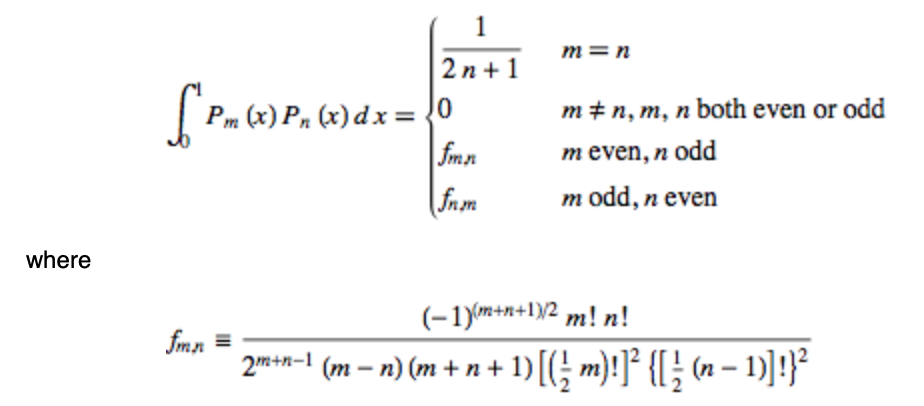# Legendre Polynomial Integral over half space

I need to compute the following integral $$I_{n,m} := \int_0^1 P_n(x) P_m(x) \; \mathrm{d}x$$ where $$P_n$$ is the Legendre polynomial.

For an even sum $$n+m=2l$$ it is easy to show that $$I_{n,m} = \frac{1}{2} \int_{-1}^1 P_n(x) P_m(x) \; \mathrm{d}x = \delta_{n,m} \frac{1}{2n+1} \,.$$

A length calculation arises for an odd sum $$n+m=2l+1$$. Has someone finished the calculation?

## 3 Answers

Integration of Equation (34) in MathWorld gives the integral $$I_{nm}$$ as a sum $$I_{nm}=\sum _{q=0}^m \frac{2^{-q}}{q+1} \binom{-m-1}{q} \binom{m}{q} \, _3F_2\left(-n,n+1,q+1;1,q+2;\tfrac{1}{2}\right).$$

As noted by the OP, Mathworld also gives the explicit expression:• Thank you! The link to MAthWorld was really helpful as there is an easy/explicit solution given there. Look to equation (51,52) or follow those links oeis.org/A078297 oeis.org/A078298 – jack Jul 20 at 16:13
• how could I have missed that! (I added the equations 51,51 for reference) – Carlo Beenakker Jul 20 at 18:19

I found the following answer, based on the idea by

Dougall, John, The product of two Legendre polynomials, Proc. Glasg. Math. Assoc. 1, 121-125 (1953). ZBL0052.06404.

Expand the product in Legendre basis $$P_n(x) P_m(x) = \sum_{k=0}^l A_{2k} P_{2l+1-2k}(x) \\ \quad \text{for} \quad A_{2k} = \frac{4(l-k)+3}{2(2l-k)+3} \frac{\lambda_k \lambda_{n-k} \lambda_{m-k}}{\lambda_{2l+1-k}} \,, \lambda_k = \frac{(2k)!}{2^{n+1} n!} \,.$$ Integration is now trivial $$I_{n,m} = \sum_{k=0}^l A_{2k} \int_0^1 P_{2l+1-2k}(x) \, \mathrm{d}x \\ \int_0^1 P_{2p+1}(x) \, \mathrm{d}x = \frac{(-1)^p}{4^{p+1}} \frac{(2p)!}{(p!)^2} \frac{2}{p+1}$$

Experimentally, $$12(-1)^{k+1}2^{2k}I_{1,2k}$$ matches the super ballot numbers and $$2^{2k+2}I_{k,k+1}$$ matches A018224.

• Thank you for the feedback but this does not answer my question. I asked for general solutions. – jack Jul 20 at 16:06